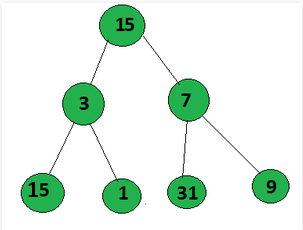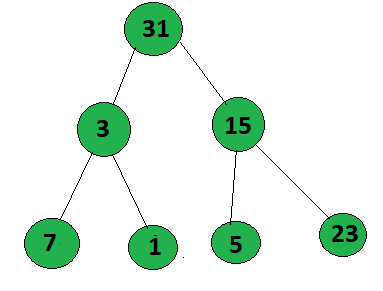# Find maximum GCD value from root to leaf in a Binary tree

Given a Binary Tree, the task is to find the maximum value of GCD from any path from the root node to the leaf node.

Examples:

Input: Below is the given tree:Output: 3
Explanation:
Path 1: 15->3->5 = gcd(15, 3, 15) =3
Path 2: 15->3->1 =gcd(15, 3, 1) = 1
Path 3: 15->7->31=gcd(15, 7, 31)= 1
Path 4: 15->7->9 = gcd(15, 7, 9) =1, out of these 3 is the maximum.

Input: Below is the given tree:Output: 1

Approach: The idea is to traverse all the paths from the root node to the leaf node and calculate the GCD of all the nodes that occurred in that path. Below are the steps:

1. Perform a preorder traversal on the given Binary Tree.
2. Iterate over all the paths and track all path values in an array.
3. Whenever encountered a leaf value then find the GCD of all the values in an array.
4. Update the GCD to the maximum value.

Below is the implementation of the above approach:

## C++

 `// C++ program for the above approach` `#include ` `using` `namespace` `std;`   `// Initialise to update the maximum` `// gcd value from all the path` `int` `maxm = 0;`   `// Node structure` `struct` `Node {` `    ``int` `val;`   `    ``// Left & right child of the node` `    ``Node *left, *right;`   `    ``// Intialize consutructor` `    ``Node(``int` `x)` `    ``{` `        ``val = x;` `        ``left = NULL;` `        ``right = NULL;` `    ``}` `};`   `// Function to find gcd of a and b` `int` `gcd(``int` `a, ``int` `b)` `{` `    ``if` `(b == 0)` `        ``return` `a;` `    ``return` `gcd(b, a % b);` `}`   `// function to find the gcd of a path` `int` `find_gcd(vector<``int``> arr)` `{` `    ``if` `(arr.size() == 1)` `        ``return` `arr;`   `    ``int` `g = arr;`   `    ``for` `(``int` `i = 1; i < arr.size(); i++) {` `        ``g = gcd(g, arr[i]);` `    ``}`   `    ``return` `g;` `}`   `// Function to find the maximum value` `// of gcd from root to leaf` `// in a Binary tree` `void` `maxm_gcd(Node* root, vector<``int``> ans)` `{` `    ``// Check if root is not null` `    ``if` `(!root)` `        ``return``;`   `    ``if` `(root->left == NULL` `        ``and root->right == NULL) {` `        ``ans.push_back(root->val);`   `        ``// Find the maxmimum gcd of` `        ``// path value and store in` `        ``// global maxm varibale` `        ``maxm = max(find_gcd(ans),` `                   ``maxm);`   `        ``return``;` `    ``}`   `    ``// Traverse left of binary tree` `    ``ans.push_back(root->val);` `    ``maxm_gcd(root->left, ans);`   `    ``// Traverse right of the binary tree` `    ``maxm_gcd(root->right, ans);` `}`   `// Driver Code` `int` `main()` `{` `    ``// Given Tree` `    ``Node* root = ``new` `Node(15);` `    ``root->left = ``new` `Node(3);` `    ``root->right = ``new` `Node(7);` `    ``root->left->left = ``new` `Node(15);` `    ``root->left->right = ``new` `Node(1);` `    ``root->right->left = ``new` `Node(31);` `    ``root->right->right = ``new` `Node(9);`   `    ``// Function Call` `    ``maxm_gcd(root, {});`   `    ``// Print the maximum AND value` `    ``cout << maxm << endl;`   `    ``return` `0;` `}`

## Java

 `// Java program for the above approach` `import` `java.util.*;`   `class` `GFG{`   `// Initialise to update the maximum` `// gcd value from all the path` `static` `int` `maxm = ``0``;`   `// Node structure` `static` `class` `Node ` `{` `    ``int` `val;`   `    ``// Left & right child of the node` `    ``Node left, right;`   `    ``// Intialize consutructor` `    ``Node(``int` `x)` `    ``{` `        ``val = x;` `        ``left = ``null``;` `        ``right = ``null``;` `    ``}` `};`   `// Function to find gcd of a and b` `static` `int` `gcd(``int` `a, ``int` `b)` `{` `    ``if` `(b == ``0``)` `        ``return` `a;` `    ``return` `gcd(b, a % b);` `}`   `// Function to find the gcd of a path` `static` `int` `find_gcd(Vector arr)` `{` `    ``if` `(arr.size() == ``1``)` `        ``return` `arr.get(``0``);`   `    ``int` `g = arr.get(``0``);`   `    ``for``(``int` `i = ``1``; i < arr.size(); i++)` `    ``{` `        ``g = gcd(g, arr.get(``1``));` `    ``}` `    ``return` `g;` `}`   `// Function to find the maximum value` `// of gcd from root to leaf` `// in a Binary tree` `static` `void` `maxm_gcd(Node root, ` `                     ``Vector ans)` `{` `    `  `    ``// Check if root is not null` `    ``if` `(root == ``null``)` `        ``return``;`   `    ``if` `(root.left == ``null` `&& ` `        ``root.right == ``null``) ` `    ``{` `        ``ans.add(root.val);`   `        ``// Find the maxmimum gcd of` `        ``// path value and store in` `        ``// global maxm varibale` `        ``maxm = Math.max(find_gcd(ans),` `                        ``maxm);` `                        `  `        ``return``;` `    ``}`   `    ``// Traverse left of binary tree` `    ``ans.add(root.val);` `    ``maxm_gcd(root.left, ans);`   `    ``// Traverse right of the binary tree` `    ``maxm_gcd(root.right, ans);` `}`   `// Driver Code` `public` `static` `void` `main(String[] args)` `{` `    `  `    ``// Given Tree` `    ``Node root = ``new` `Node(``15``);` `    ``root.left = ``new` `Node(``3``);` `    ``root.right = ``new` `Node(``7``);` `    ``root.left.left = ``new` `Node(``15``);` `    ``root.left.right = ``new` `Node(``1``);` `    ``root.right.left = ``new` `Node(``31``);` `    ``root.right.right = ``new` `Node(``9``);`   `    ``// Function call` `    ``maxm_gcd(root, ``new` `Vector<>());`   `    ``// Print the maximum AND value` `    ``System.out.print(maxm + ``"\n"``);` `}` `}`   `// This code is contributed by Rajput-Ji`

## Python3

 `# Python3 implementation of` `# the above approach`   `# Initialise to update the maximum` `# gcd value from all the path` `global` `maxm` `maxm ``=` `0`   `# Node structure` `class` `Node:`   `    ``# Intialize consutructor` `    ``def` `__init__(``self``, x):`   `        ``self``.val ``=` `x` `        ``self``.left ``=` `None` `        ``self``.right ``=` `None`   `# Function to find gcd of a and b` `def` `gcd(a, b):`   `    ``if``(b ``=``=` `0``):` `        ``return` `a` `    ``return` `gcd(b, a ``%` `b)`   `# Function to find the gcd of a path` `def` `find_gcd(arr):`   `    ``if``(``len``(arr) ``=``=` `1``):` `        ``return` `arr[``0``]`   `    ``g ``=` `arr[``0``]`   `    ``for` `i ``in` `range``(``1``, ``len``(arr)):` `        ``g ``=` `gcd(g, arr[i])`   `    ``return` `g`   `# Function to find the maximum value` `# of gcd from root to leaf` `# in a Binary tree` `def` `maxm_gcd(root, ans):`   `    ``global` `maxm`   `    ``# Check if root is not null` `    ``if``(``not` `root):` `        ``return`   `    ``if``(root.left ``=``=` `None` `and` `       ``root.right ``=``=` `None``):` `        ``ans.append(root.val)`   `        ``# Find the maxmimum gcd of` `        ``# path value and store in` `        ``# global maxm varibale` `        ``maxm ``=` `max``(find_gcd(ans), maxm)`   `        ``return`   `    ``# Traverse left of binary tree` `    ``ans.append(root.val)` `    ``maxm_gcd(root.left, ans)`   `    ``# Traverse right of the binary tree` `    ``maxm_gcd(root.right, ans)`   `# Driver Code` `if` `__name__ ``=``=` `'__main__'``:`   `    ``# Given Tree` `    ``root ``=` `Node(``15``)` `    ``root.left ``=` `Node(``3``)` `    ``root.right ``=` `Node(``7``)` `    ``root.left.left ``=` `Node(``15``)` `    ``root.left.right ``=` `Node(``1``)` `    ``root.right.left ``=` `Node(``31``)` `    ``root.right.right ``=` `Node(``9``)`   `    ``# Function call` `    ``maxm_gcd(root, [])` `    `  `    ``# Print the maximum AND value` `    ``print``(maxm)`   `# This code is contributed by Shivam Singh`

## C#

 `// C# program for the above approach` `using` `System;` `using` `System.Collections.Generic;` `class` `GFG{`   `// Initialise to update the maximum` `// gcd value from all the path` `static` `int` `maxm = 0;`   `// Node structure` `class` `Node ` `{` `    ``public` `int` `val;`   `    ``// Left & right child of the node` `    ``public` `Node left, right;`   `    ``// Intialize consutructor` `    ``public` `Node(``int` `x)` `    ``{` `        ``val = x;` `        ``left = ``null``;` `        ``right = ``null``;` `    ``}` `};`   `// Function to find gcd of a and b` `static` `int` `gcd(``int` `a, ``int` `b)` `{` `    ``if` `(b == 0)` `        ``return` `a;` `    ``return` `gcd(b, a % b);` `}`   `// Function to find the gcd of a path` `static` `int` `find_gcd(List<``int``> arr)` `{` `    ``if` `(arr.Count == 1)` `        ``return` `arr;`   `    ``int` `g = arr;`   `    ``for``(``int` `i = 1; i < arr.Count; i++)` `    ``{` `        ``g = gcd(g, arr);` `    ``}` `    ``return` `g;` `}`   `// Function to find the maximum value` `// of gcd from root to leaf` `// in a Binary tree` `static` `void` `maxm_gcd(Node root, ` `                     ``List<``int``> ans)` `{` `    `  `    ``// Check if root is not null` `    ``if` `(root == ``null``)` `        ``return``;`   `    ``if` `(root.left == ``null` `&& ` `        ``root.right == ``null``) ` `    ``{` `        ``ans.Add(root.val);`   `        ``// Find the maxmimum gcd of` `        ``// path value and store in` `        ``// global maxm varibale` `        ``maxm = Math.Max(find_gcd(ans),` `                                 ``maxm);` `                        `  `        ``return``;` `    ``}`   `    ``// Traverse left of binary tree` `    ``ans.Add(root.val);` `    ``maxm_gcd(root.left, ans);`   `    ``// Traverse right of the binary tree` `    ``maxm_gcd(root.right, ans);` `}`   `// Driver Code` `public` `static` `void` `Main(String[] args)` `{` `    `  `    ``// Given Tree` `    ``Node root = ``new` `Node(15);` `    ``root.left = ``new` `Node(3);` `    ``root.right = ``new` `Node(7);` `    ``root.left.left = ``new` `Node(15);` `    ``root.left.right = ``new` `Node(1);` `    ``root.right.left = ``new` `Node(31);` `    ``root.right.right = ``new` `Node(9);`   `    ``// Function call` `    ``maxm_gcd(root, ``new` `List<``int``>());`   `    ``// Print the maximum AND value` `    ``Console.Write(maxm + ``"\n"``);` `}` `}`   `// This code is contributed by sapnasingh4991`

Output:

```3

```

Time Complexity: O(N2)
Auxiliary Space: O(N)

Attention reader! Don’t stop learning now. Get hold of all the important DSA concepts with the DSA Self Paced Course at a student-friendly price and become industry ready.

My Personal Notes arrow_drop_upCheck out this Author's contributed articles.

If you like GeeksforGeeks and would like to contribute, you can also write an article using contribute.geeksforgeeks.org or mail your article to contribute@geeksforgeeks.org. See your article appearing on the GeeksforGeeks main page and help other Geeks.

Please Improve this article if you find anything incorrect by clicking on the "Improve Article" button below.